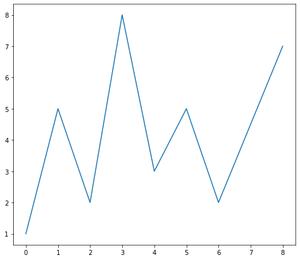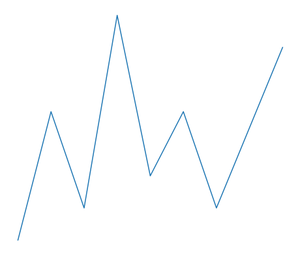Related Articles
How to remove the frame from a Matplotlib figure in Python?
• Last Updated : 11 Dec, 2020

A frame in the Matplotlib figure is an object inside which given data is represented using independent Axes. These axes represent left, bottom, right and top which can be visualized by Spines(lines) and ticks.

To remove the frame (box around the figure) in Matplotlib we follow the steps. Here firstly we will plot a 2D figure in Matplotlib by importing the Matplotlib library.

Syntax: plt.tick_params(axis=’x’, which=’both’, bottom=False, top=False, labelbottom=False)

Approach:

• Select the axis to be applied. And select the tick by which the parameter are applied generally it can be ‘major’ , ‘minor’ or ‘both’.
• Get the current Axex and select the spines’ visibility as False.

## Python3

 `# Importing the Library`` ` `import` `matplotlib.pyplot as plt``# Defining X-axis and Y-axis data Points``x ``=` `[``0``, ``1``, ``2``, ``3``, ``4``, ``5``, ``6``, ``8``]``y ``=` `[``1``, ``5``, ``2``, ``8``, ``3``, ``5``, ``2``, ``7``]`` ` `# Defining the Width and height of the Figure``plt.figure(figsize``=``(``8``, ``7``))``plt.plot(x, y)``plt.show()`

Output:The given output contains the frame with the spines and ticks. By means of removing the frame we are actually removing the box around the figure containing axes( left, bottom, right, Top).
To remove the spines denoted by Black Lines we can follow the steps,

## Python3

 `plt.figure(figsize``=``(``4``, ``3``))`` ` `plt.plot(x, y)`` ` `# Selecting the axis-X making the bottom and top axes False.``plt.tick_params(axis``=``'x'``, which``=``'both'``, bottom``=``False``,``                ``top``=``False``, labelbottom``=``False``)`` ` `# Selecting the axis-Y making the right and left axes False``plt.tick_params(axis``=``'y'``, which``=``'both'``, right``=``False``,``                ``left``=``False``, labelleft``=``False``)`` ` `# Iterating over all the axes in the figure``# and make the Spines Visibility as False``for` `pos ``in` `[``'right'``, ``'top'``, ``'bottom'``, ``'left'``]:``    ``plt.gca().spines[pos].set_visible(``False``)``plt.show()`

Output:Attention geek! Strengthen your foundations with the Python Programming Foundation Course and learn the basics.

To begin with, your interview preparations Enhance your Data Structures concepts with the Python DS Course.

My Personal Notes arrow_drop_up